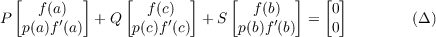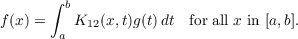#### Vol. 18, No. 1, 1966

 Download this articleFor screen For printingRecent Issues Vol. 325: 1  2 Vol. 324: 1  2 Vol. 323: 1  2 Vol. 322: 1  2 Vol. 321: 1  2 Vol. 320: 1  2 Vol. 319: 1  2 Vol. 318: 1  2Online Archive Volume: Issue:The Journal Subscriptions Editorial Board Officers Contacts Submission Guidelines Submission Form Policies for Authors ISSN: 1945-5844 (e-only) ISSN: 0030-8730 (print) Special Issues Author Index To Appear Other MSP Journals
The lack of self-adjointness in three-point boundary value problems

### John William Neuberger

Vol. 18 (1966), No. 1, 165–168
##### Abstract

Suppose that a < c < b, C[a,b] is the set of all real-valued continuous functions on [a,b], each of p and q is in C[a,b], p(x) > 0 for all x in [a,b] and each of P, Q and S is a real 2 ×2 matrix. The assumption is made that the only member f of C[a,b] so that (pf)′− qf = 0 andis the zero function. It follows that there is a real-valued continuous function K12 on [a,b] × [a,b] such that if g is in C[a,b], then the only element f of C[a,b] so that (pf)′− qf = g and (Δ) holds is given byIn this note it is shown that if in addition it is specified that Q is not the zero 2 ×2 matrix, then K12 is not symmetric, i.e., it is not true that K12(x,t) = K12(t,x) for all x, t in [a,b].

Primary: 34.36
##### Milestones